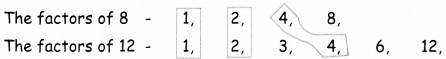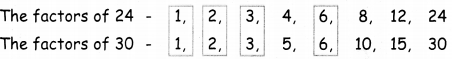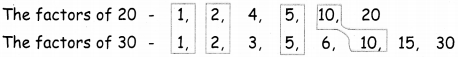Students can download 5th Maths Term 2 Chapter 2 Numbers Ex 2.1 Questions and Answers, Notes, Samacheer Kalvi 5th Maths Guide Pdf helps you to revise the complete Tamilnadu State Board New Syllabus, helps students complete homework assignments and to score high marks in board exams.

## Tamilnadu Samacheer Kalvi 5th Maths Solutions Term 2 Chapter 2 Numbers Ex 2.1

Question 1.
Find the common factors for:
(i) 8 and 12
(ii) 24 and 30
(iii) 20 and 30
(i) 8 and 12The common factors of 8, 12 are 1, 2, 4(ii) 24 and 30The common factors are 24, 30 are 1, 2, 3, 6(iii) 20 and 30The common factors of 20, 30 are 1, 2, 5, 10